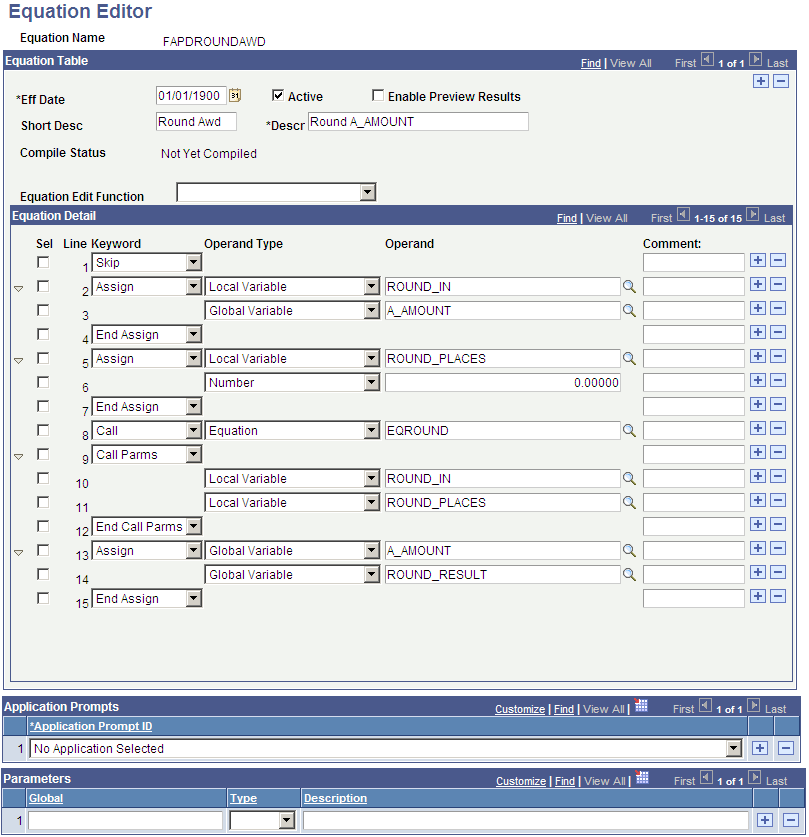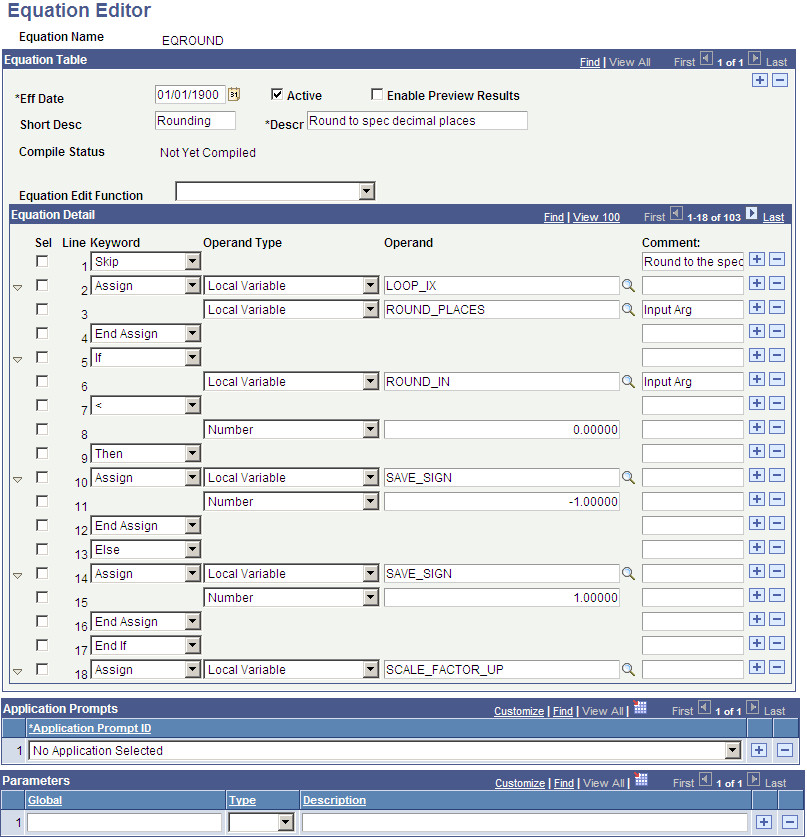Rounding in PeopleSoft Financial Aid Packaging Using Equations

This section provides an overview of rounding in Financial Aid Packaging.

The Packaging routine does not round up to whole dollar amounts when your equation yields a decimal result for a packaging limit. If the result contains decimals, the Packaging routine produces the following error message: "Message # 9146 Cannot disburse in whole dollars; award contains cents."

For those equations that need to yield a whole dollar result, you must use a rounding equation. You could accomplish this by employing one of two options:

• Option 1

1. Create your A_AMOUNT equation, and at the bottom of the equation insert a Call statement to the delivered sample equation FAPDROUNDAWD.

2. Review the FAPDROUNDAWD equation to determine the number of places beyond the decimal point that you want (0=integers or whole numbers, 1, 2, and so forth).

3. Ensure that the Equation EQROUND is called as part of FAPDROUNDAWD.

• Option 2

1. Create your A_AMOUNT equation, and at the bottom of the equation insert a Call statement to the delivered sample equation FAPDROUNDAWD.

2. Review the FAPDROUNDAWD equation to determine the number of places beyond the decimal point that you want (0=integers or whole numbers, 1, 2, and so forth).

3. Ensure that the Equation EQROUND is called as part of FAPDROUNDAWD.

Important! For this rounding logic to work properly, all elements of FAPDROUNDAWD must be employed, and EQROUND must be called as part of that routine. Do not modify these components.

Here are two of the rounding equations that PeopleSoft Campus Solutions delivers:

Image: Equation Editor (FAPDROUNDAWD) page

This example illustrates the fields and controls on the Equation Editor (FAPDROUNDAWD) page. You can find definitions for the fields and controls later on this page.Image: Equation Editor (EQROUND) page

This example illustrates the fields and controls on the Equation Editor (EQROUND) page. You can find definitions for the fields and controls later on this page.